## Introduction

We assume you went through the previous vignettes that showed you how to use the functionality provided by flowdiagramr to produce fairly good looking (in our biased opinion) diagrams. We believe (hope) that this often gives you a diagram you are happy with.

However, occasionally you might want to make additional tweaks beyond what flowdiagramr can do. Fortunately for you, there is a way to do this, which is the topic of this vignette. There’s one easy way, and one approach that’s a bit more involved but also more flexible.

## Modifying the ggplot object

The object returned by make_diagram() is a ggplot object, which means you can do all the things you can do to those objects. That includes further alterations and updates. Here are a few simple examples.

### Example 1

Back to our simple SIR model

# specify the model
variables = c("S","I","R")
flows = list(S_flows = c("n", "-b*S*I", "-m*S"),
I_flows = c("+b*S*I","-g*I", "-m*I"),
R_flows = c("g*I", "-m*R"))
sirmodel = list(variables = variables, flows = flows)
# prepare, make and plot
diag_list <- prepare_diagram(sirmodel)
diag1 <- make_diagram(diag_list)
plot(diag1)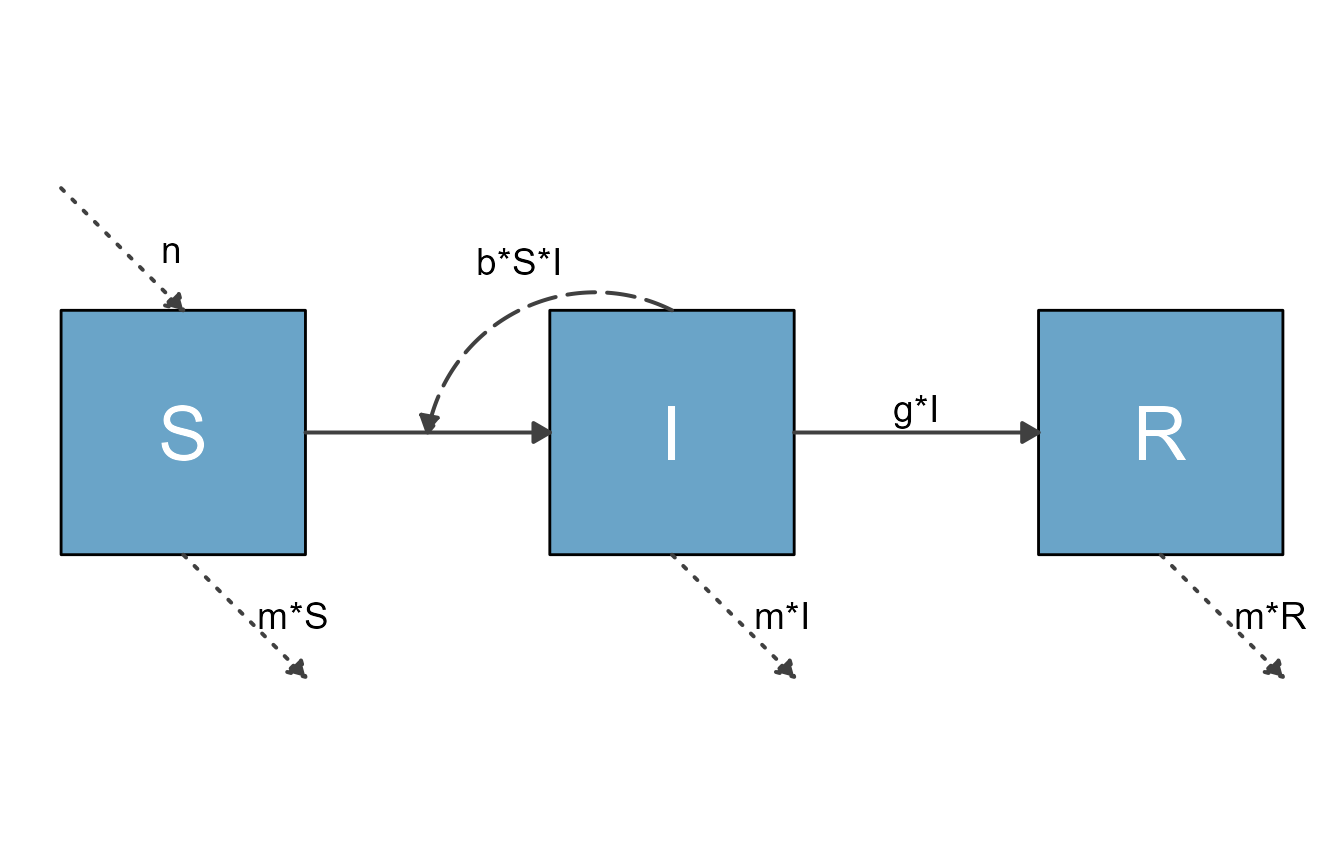Let’s assume you want to give it a title and add some more text into the plot. You can just use the regular ggplot2 syntax.

diag2 <- diag1 + ggtitle("My diagram") + geom_label(aes(x=2,y=2),label="My label")
plot(diag2)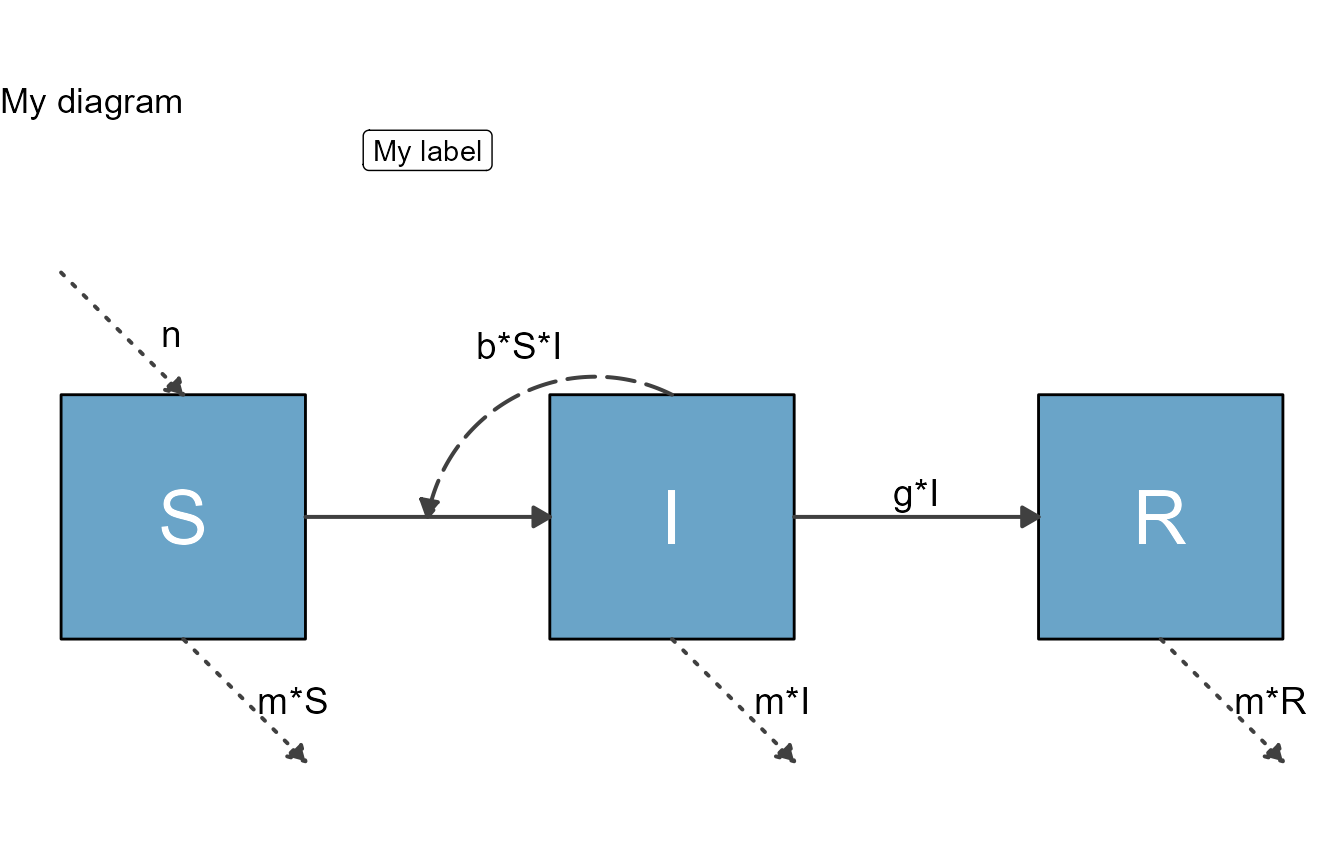### Example 2

Here is another, somewhat more involved example. It shows the overall suggested workflow, namely first using all the functionality that flowdiagramr provides, and then further modifying the plot with ggplot2 commands.

For this one, we return to the big 7-compartment model we’ve been exploring in previous vignettes.

Here is what we did previously.

Define model:

variables = c("Sc","Ic","Rc","Sa","Ia","Ra","P")
flows = list(Sc_flows = c("-bcc*Sc*Ic","-bca*Sc*Ia","-bcp*Sc*P"),
Ic_flows = c("bcc*Sc*Ic","bca*Sc*Ia","bcp*Sc*P","-gc*Ic"),
Rc_flows = c("gc*Ic"),
Sa_flows = c("-bac*Sa*Ic","-baa*Sa*Ia","-bap*Sa*P"),
Ia_flows = c("bac*Sa*Ic","baa*Sa*Ia","bap*Sa*P","-ga*Ia"),
Ra_flows = c("ga*Ia"),
P_flows = c("rc*Ic","ra*Ia","-d*P")
)
mymodel = list(variables, flows)

Define layout:

mysettings = list( varlocations = matrix(data = c("Sc", "", "Ic", "Rc",
"",   "P", "",   "",
"Sa", "", "Ia", "Ra"),nrow = 3, byrow = TRUE),
varspace_x_size = 2,
varspace_y_size = 1
)

## Modifying the ggplot2 code

While the above approach toward modifying the ggplot object returned by make_diagram() gives you some additional flexibility, you can go a step further. The most flexible approach to adjust diagrams is to modify the ggplot code that produces the diagram. flowdiagramr provides a function that returns the code, which you can then edit like you would any ggplot code. We’ll illustrate this approach with a few examples.

### Example 1

# specify the model
variables = c("S","I","R")
flows = list(S_flows = c("n", "-b*S*I", "-m*S"),
I_flows = c("+b*S*I","-g*I", "-m*I"),
R_flows = c("g*I", "-m*R"))
sirmodel = list(variables = variables, flows = flows)

Next, you prepare the model

diag_list <- prepare_diagram(sirmodel)

Once you have the diagram list ready, you can send it to write_diagram, a function that writes the full code needed to generate the diagram. You can specify the location and name for the file. If you don’t provide those, it will be stored in the current working directory under the name diagram_code.R.

# write the current diagram code to a file, overwrite if it exists
write_diagram(diagram_list = diag_list, filename = 'model1_diagram_code.R', always_overwrite = TRUE)

If things worked well, you should get a message telling you the location where the code was saved. The code that is generated is completely self-contained, that means you can give it to anyone, who can run it and reproduce your model figure.

If you run the code that is generated, it will create the diagram structure as an object called diagram_plot. You can then plot or save that object (there is code at the bottom of the generated file for that).

For this example, here is what you get.

source('model1_diagram_code.R')
plot(diagram_plot)Having all the code that generates the figure allows you to do some fine-tuning. We provided hopefully enough comments in the code to make it clear what happens where. That said, to be able to tweak the diagram this way requires being familiar with creating figures in ggplot.

Let’s assume for this example that you want to add some text below the I compartment. In this case, you don’t need to modify any of the existing code, just add a some code at the end. You could do this using the approach described above, without editing the R code. But here we’ll do it by editing the code.

The following lines of code appended to the bottom of sirmodel1_diagram_code.R, just before the final plot statement, will do the trick:

#######################
### LAST PART OF THE GENERATED CODE BEFORE ADDITION
#######################
if(with_grid == FALSE) {
diagram_plot <- diagram_plot +
theme_void()
} else {
# The else here may seem silly, but otherwise the returned plot is NULL
diagram_plot <- diagram_plot
}

#######################
#######################
# make a new data frame of text
text_df <- data.frame(  x = 3,  y = -1,
lab = "Infected and Infectious"
)
diagram_plot <- diagram_plot +
geom_text(data = text_df, aes(x = x, y = y, label = lab), size = 8)

#######################
### PART OF THE GENERATED CODE AFTER ADDITION
#######################
# These lines plot or save the generated diagram.
# Uncomment them if you want to perform either action.
# plot(diagram_plot)
# ggsave('diagram_plot.png',diagram_plot)

Then save the new code, let’s call it sirmodel1_diagram_code_modified.R. Running the new code will add the wanted text to the diagram plot

source('model1_diagram_code_modified.R')
plot(diagram_plot)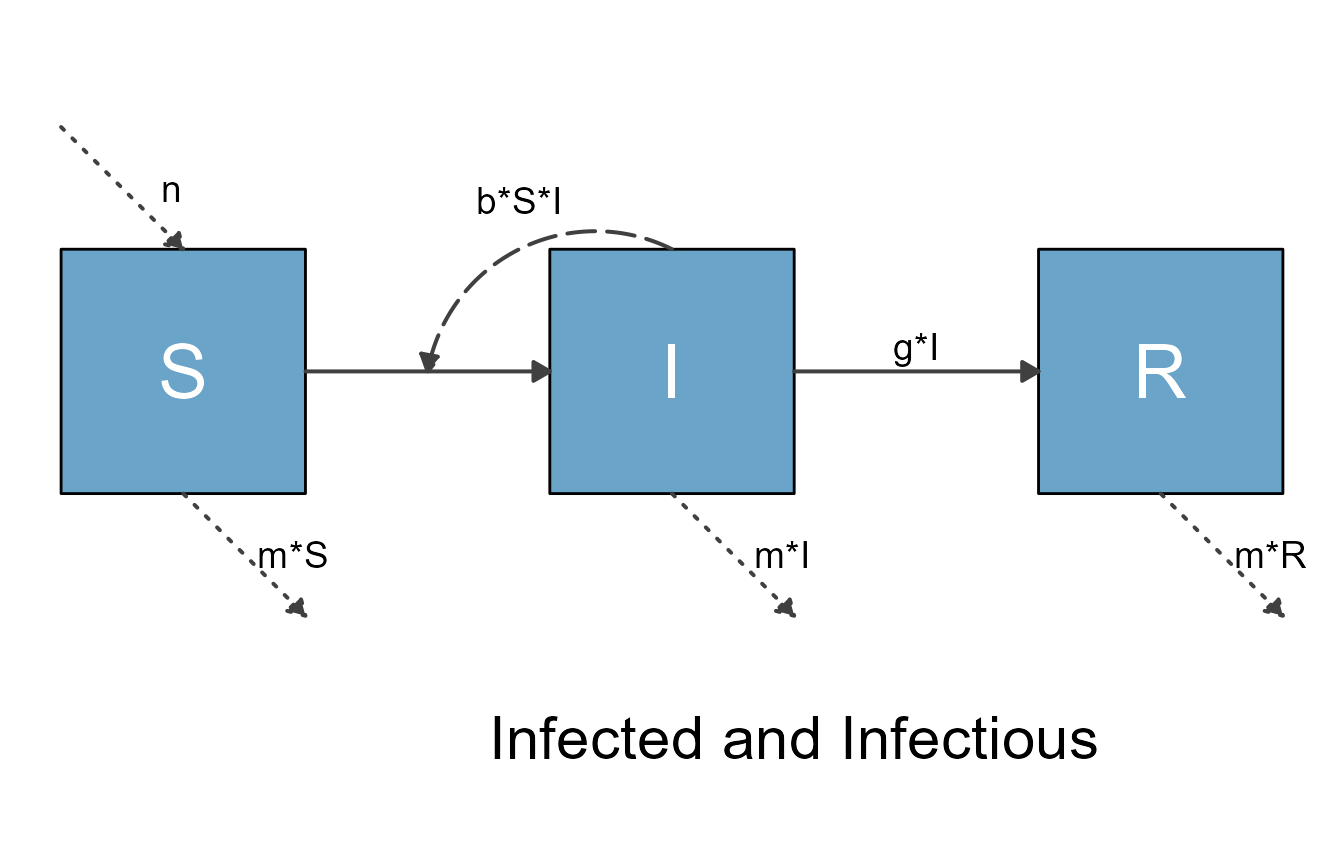### Example 2

Let’s look at another example, one that requires this approach. In general, it is advisable to do as much styling and adjusting as possible using the flowdiagramr functions. If that doesn’t get you all the way, use the option described at the beginning of the vignette, or produce the code and edit it.

Here is an example showing how to use the different customization options. For this example, we revisit our somewhat complex 7-compartment model. This is all the code we had previously to get a decent figure:

variables = c("Sc","Ic","Rc","Sa","Ia","Ra","P")
flows = list(Sc_flows = c("-bcc*Sc*Ic","-bca*Sc*Ia","-bcp*Sc*P"),
Ic_flows = c("bcc*Sc*Ic","bca*Sc*Ia","bcp*Sc*P","-gc*Ic"),
Rc_flows = c("gc*Ic"),
Sa_flows = c("-bac*Sa*Ic","-baa*Sa*Ia","-bap*Sa*P"),
Ia_flows = c("bac*Sa*Ic","baa*Sa*Ia","bap*Sa*P","-ga*Ia"),
Ra_flows = c("ga*Ia"),
P_flows = c("rc*Ic","ra*Ia","-d*P")
)
mymodel = list(variables, flows)
mysettings = list( varlocations = matrix(data = c("Sc", "", "Ic", "Rc",
"",   "P", "",   "",
"Sa", "", "Ia", "Ra"),nrow = 3, byrow = TRUE),
varspace_x_size = 2,
varspace_y_size = 1
)

Prepare the diagram with additional settings.

diagram_list <- prepare_diagram(mymodel, mysettings)

Apply styling

# define list of updates
diagram_settings = list(var_fill_color = c(Sc = "#cde9fa", Ic = "#cde9fa",
Rc = "#cde9fa", Sa = "#40f9cf",
Ia = "#40f9cf", Ra = "#40f9cf",
P = "#b2b2b2"),
var_outline_color = c(all = "#031e79"),
var_label_size = c(all = 8),
var_label_color = c(all = "black"),
flow_curvature = c(i_baaSaIa = -0.5, i_bccScIc = 0.5,
i_bacSaIc = -0.3, i_bcaScIa = 0.3,
i_raIa = 0, i_rcIc = 0, i_bcpScP = -0.5),
flow_xstart = c(i_bcpScP = -0.5, i_bapSaP = -0.5),
flow_ystart = c(i_bcpScP = -0.5, i_bapSaP = -0.5, i_bacSaIc = -1),
flow_line_color = c(interaction = "#f94075",
external = "gray",
i_baaSaIa = "#40f9cf", i_bacSaIc = "#cde9fa",
i_bapSaP = "orange", i_bcpScP = "orange",
i_bccScIc = "#cde9fa", i_bcaScIa = "#40f9cf"),
flow_line_size = c(all = 1.25),
flow_xlabel = c(i_bcaScIa = 0.75, i_bacSaIc = 3.5,
i_bapSaP = -0.75, i_bcpScP = -2,
i_raIa = -0.25, i_rcIc = 0.5),
flow_ylabel = c(i_bcaScIa = -2.5, i_bacSaIc = -0.25,
i_baaSaIa = -2, e_dP = -0.5,
i_rcIc = -0.75,m_gcIc = 0.25, m_gaIa = 0.25)
)
diagram_list2 <- update_diagram(diagram_list, diagram_settings) 

Make diagram

diag <- make_diagram(diagram_list2)

Now, export the R code.

write_diagram(diagram_list = diagram_list2,
filename = 'model2_diagram_code.R',
always_overwrite = TRUE)

If you run the code that is generated, you should see a figure like this

source('model2_diagram_code.R')
plot(diagram_plot)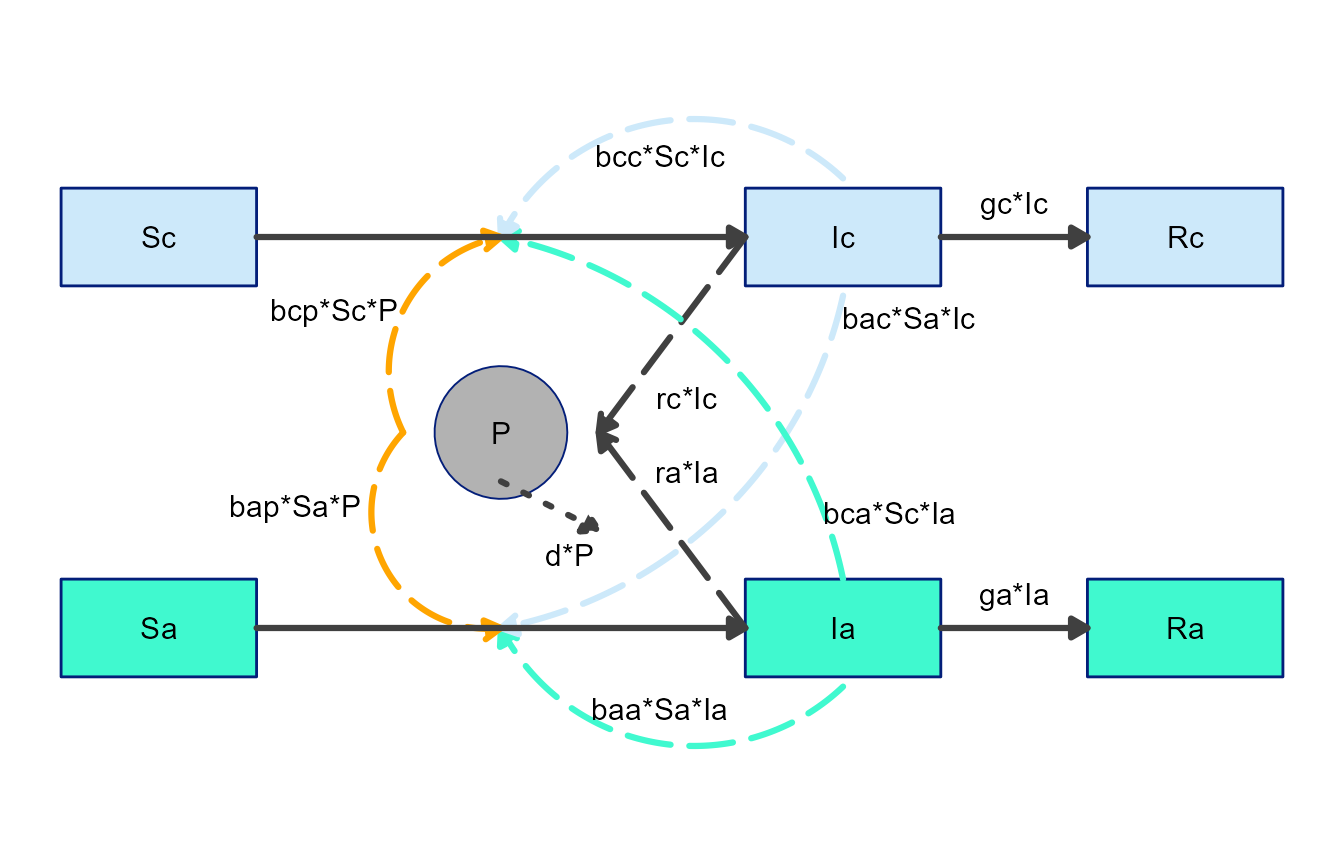Let’s assume that you are mostly happy with this figure, but you want to make another tweak. This time, you want to turn the shape of the P box into a circle.

You can find the part of the code that creates the boxes (it’s the first for loop) and modify as follows:

#######################
### LAST PART OF THE GENERATED CODE BEFORE ADDITION
#######################
for(i in 1:nrow(variables)) {

#######################
#######################
if(variables\$name[i] == "P") {
diagram_plot <- diagram_plot +
geom_point(
data = variables[i, ],
aes(x = xmin+(xmax-xmin)/2, y = ymin+(ymax-ymin)/2), #centers
color = variables[i, "outline_color"],
fill = variables[i, "fill_color"],
shape = 21,
size = 23
)
} else {
diagram_plot <- diagram_plot +
geom_rect(
data = variables[i, ],
aes(xmin = xmin, xmax = xmax, ymin = ymin, ymax = ymax),
color = variables[i, "outline_color"],
fill = variables[i, "fill_color"]
)
}

Now save the modified script, source it and plot the generated diagram_plot object to get this figure:

source('model2_diagram_code_modified.R')
plot(diagram_plot)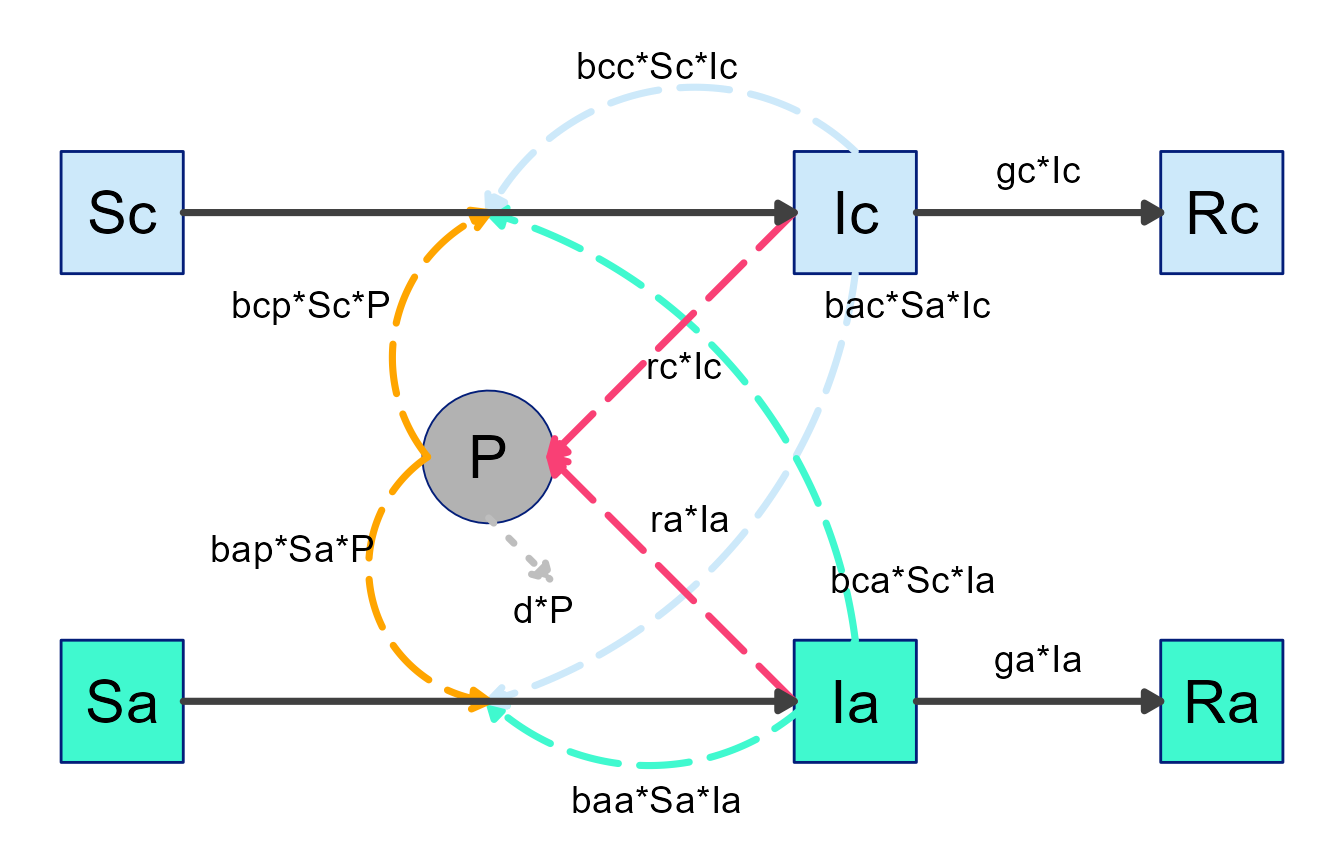## General suggestions

It should be clear from these examples that once you have the ggplot code, you can do any modifications you want. At this point, the options are endless and you can change the code to get exactly what you want - at the expense of having to write/edit code yourself.

As mentioned before, the best workflow is to use the flowdiagramr functionality to get as far as it takes you, and then as needed make further manual modifications.

Of course, once you have started manual editing of the code, things are not reversible, i.e. you have basically left the flowdiagramr package infrastructure and are now adjusting the diagram on your own. You can of course always re-generate the code and copy your modifications over (as long as you remember to not overwrite your code.)

## Next steps

This concludes the main tutorials for the package. Several additional vignettes are available. One shows further model examples, another one discusses additional topics such as using flowdiagramr together with our modelbuilder package and how to crop white space around the diagrams. Yet another vignette provides some examples on how to create flow diagrams for scenarios that are not based on compartmental models. It illustrates how basically anything for which you want to make a diagram consisting of nodes/boxes and flows/arrows can be created with flowdiagramr. And one more vignette compares flowdiagramr to similar R packages. You can access all those additional vignettes from the package website.